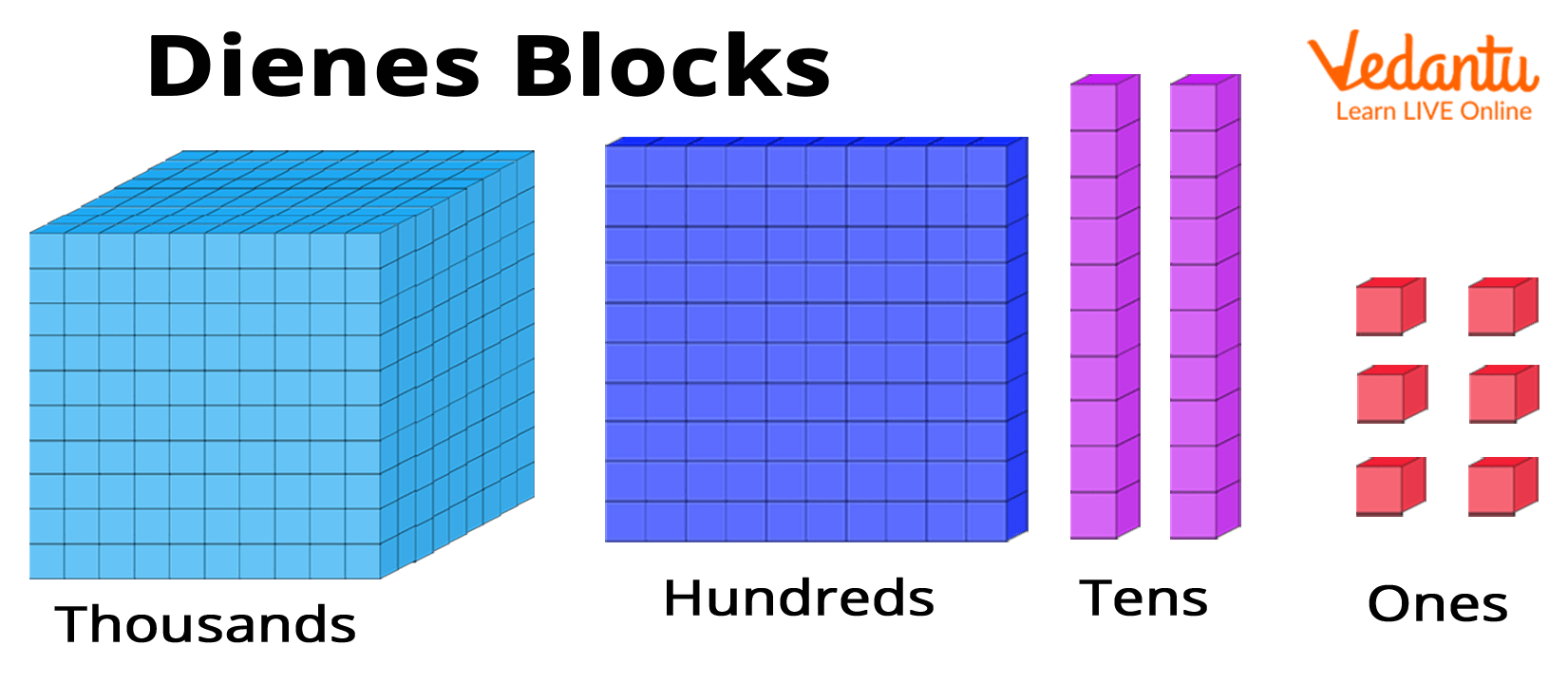Courses
Courses for Kids
Free study material
Free LIVE classes
More

# How to Teach Place Value to 5 Year Students?## Introduction to Place Value and Numbers

Kids are introduced to numbers at an early age; kids starting in Grade 2 are familiarised with the use of place value charts and their importance in writing the numbers in words. The concept of numbers and place value for Year 5 students is one of the important basics of Mathematics. The article provides an overview of the topics that are taught in fifth grade. It particularly focuses on the methods that can help teachers in teaching place value to kids in Year 5 students. Teaching place value to Year 5 students will be much easier once you go through the following.

## Fun Ways of Teaching Place Value in Year 5

Teaching new concepts is always a pleasant experience. Kids at a growing age are very curious to learn things. Mentioned below are some tips that can help teachers in teaching the new concepts like place value in year 5.

### Engaging with Kids

Learning can be a fun experience. Students and parents or teachers are equally involved in the learning process, and learning new things through activity is one of the most effective methods of engaging with the students.

One such activity can be planned using the Dienes blocks. Dienes blocks of different values can be used for place value representation. Kids can be asked to represent any number like 174 with the help of dienes. For number 174 kids would need to organise one set of 100, 7 sets of 10 and four single cubes. This can be fun and can help kids to better understand the place value chart.Dienes block used to learn place value in Year 5

### Using the Knowledge of Place Value

Parents and teachers must encourage kids to correlate the concepts of place value to other mathematical operations. This can help kids to excel academically and also improve their understanding of the concept.

In the case of place value, kids can learn about the pattern for example 4 + 3 = 7, and 0.4 + 0.3 = 0.7. The pattern that emerged here can be represented as the numbers in the calculation being ten times smaller and the total is also ten times smaller.

## Teaching Kids to Calculate Place Value in Year 5

Lastly, we must learn to calculate the place value of the numbers. We can learn it from the following examples.

Example 1. Calculate the place value of the number 24

The digit 2 in the number 24 is in the tens place, and it has a place value of 2 × 10 = 20

The digit 4 is in the one’s place, and it has the place value of 4 × 1 = 4

Example 2. Calculate the place value of 657

The place value of the digit 6 is 6 x 100 = 600

The place value of 5 in number 657 is 5 x 10 = 50

The place value of the one’s digit is 7 x 1 =7.

This was the complete discussion on methods to teach kids about place value in Year 5. We hope to have provided a better insight into the topic. An important point to note here is that it is through regular practice that a kid can enhance their skills.

Last updated date: 02nd Oct 2023
Total views: 137.1k
Views today: 2.37k

## FAQs on How to Teach Place Value to 5 Year Students?

1. What is the place value?

Place value in Mathematics can be defined as the position of a digit written in a number. It describes the position or place of a digit in a number. Every digit in a number has a distinct value depending on where it appears in the number. A number may have two digits that are similar yet have distinct values, which are determined by the digit's position in the number.

This can be explained with the following example: the number 711, the first 1 has the place value of ones or units whereas the second 1 has the palace value of tens.

2. What is the place value chart? How can help kids in understanding the concept of place value?

Place value charts assist us in ensuring that the digits are aligned correctly. A place value chart shows the proper placement of a digit in a number. Using a printable place value chart can help students memorise and solve issues on place value systems.

For each digit in a number, place values are presented in a tabular format across blank spaces on these charts. Students can directly set the digits in their respective positions according to the one in the number and evaluate their place values while referring to these charts to solve a problem.